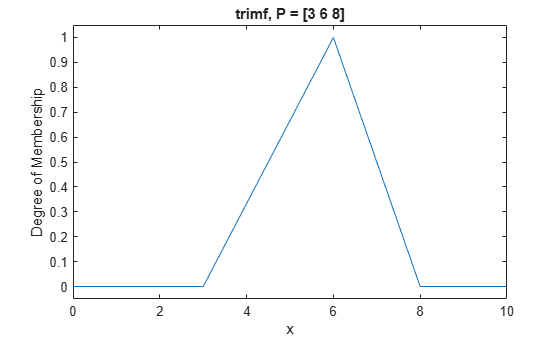trimf

Triangular membership function

Description

This function computes fuzzy membership values using a triangular membership function. You can also compute this membership function using a fismf object. For more information, see fismf Object.

This membership function is related to the trapmf membership function.

example

y = trimf(x,params) returns fuzzy membership values computed using the following triangular membership function:

$f\left(x;a,b,c\right)=\left\{\begin{array}{ll}0,\hfill & x\le a\hfill \\ \frac{x-a}{b-a},\hfill & a\le x\le b\hfill \\ \frac{c-x}{c-b},\hfill & b\le x\le c\hfill \\ 0,\hfill & c\le x\hfill \end{array}\right\}$

or, more compactly:

$f\left(x;a,b,c\right)=\mathrm{max}\left(\mathrm{min}\left(\frac{x-a}{b-a},\frac{c-x}{c-b}\right),0\right)$

To specify the parameters, a, b, and c, use params.

Membership values are computed for each input value in x.

Examples

collapse all

x = 0:0.1:10;
y = trimf(x,[3 6 8]);
plot(x,y)
xlabel('trimf, P = [3 6 8]')
ylim([-0.05 1.05])Input Arguments

collapse all

Input values for which to compute membership values, specified as a scalar or vector.

Membership function parameters, specified as the vector [a b c]. Parameters a and c define the feet of the membership function, and b defines its peak.

Output Arguments

collapse all

Membership value returned as a scalar or a vector. The dimensions of y match the dimensions of x. Each element of y is the membership value computed for the corresponding element of x.

Alternative Functionality

fismf Object

You can create and evaluate a fismf object that implements the trimf membership function.

mf = fismf("trimf",P);
Y = evalmf(mf,X);

Here, X, P, and Y correspond to the x, params, and y arguments of trimf, respectively.